# Test: Savings And Interest- 2

## 19 Questions MCQ Test Quantitative Aptitude for GMAT | Test: Savings And Interest- 2

Description
Attempt Test: Savings And Interest- 2 | 19 questions in 40 minutes | Mock test for GMAT preparation | Free important questions MCQ to study Quantitative Aptitude for GMAT for GMAT Exam | Download free PDF with solutions
QUESTION: 1

### James invested \$100,000 in fund A at the beginning of 2012. The fund made losses during the year and he suffered a loss of 20 percent. At the beginning of 2013, he took his remaining money out of fund A and invested it in fund B. Fund B gave him an annual rate of 20 percent in 2013 and 10 percent in 2014. If he did not take out the interest earned from fund B in 2013, by what percent did his money grow from the beginning of 2012 to the end of 2014?

Solution:

Given:

Fund A - 2012

• P = 100,000
• R = -20%
• T = 1 year
• Fund B – 2013 - 14
• 2013
• Principal, B = Amount in Fund A at the end of 2012
• R = 20%
• T = 1 year
• 2014
• Principal, B = Amount in Fund B at the end of 2013
• R = 10%
• T = 1 year

To Find: % Money growth from 2012 to 2014

Approach:

% Money Growth =
We know the Invested Principal in 2012
So, to answer the question, we need to find the Amount in 2014
1.
2. To find ‘Amount in 2014’, we need to know B
To know B , we need to know B . So, we’ll start working towards the

Working out: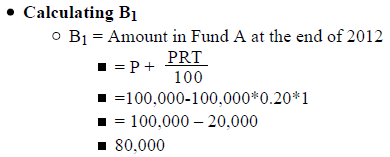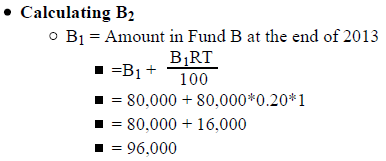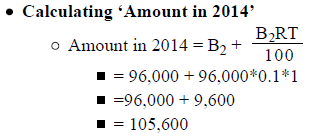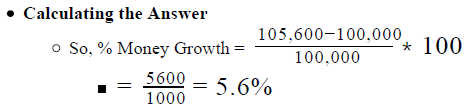Looking at the answer choices, we see that the correct answer is Option C

QUESTION: 2

### At the start of 2015, Jane opened two new accounts – X and Y – to invest her total savings of \$1000. She invested p percent of her savings in account X, which yielded a simple interest of 8 percent per annum, and the rest of the savings in account Y, which yielded a simple interest of 6 percent per annum. Was the amount of interest earned by account X greater than the amount of interest earned by account Y during the year 2015? (1) The value of p was between 25 and 30, inclusive (2) The total interest earned by the two accounts during 2015 was \$66

Solution:

Step 1 & 2: Understand Question and Draw Inference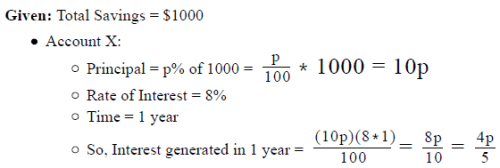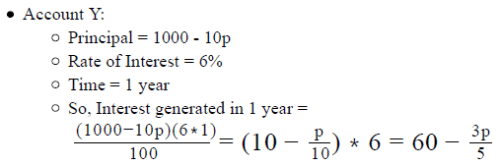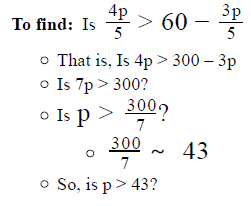Step 3 : Analyze Statement 1 independent

Statement 1 says that ‘The value of p was between 25 and 30, inclusive’

• So, the answer to the question ‘Is p > 43?’ is NO

Since Statement 1 leads to a unique answer to the question, it is sufficient

Step 4 : Analyze Statement 2 independent

Statement 2 says that ‘The total interest earned by the two accounts during 2015 was \$66’

• (Interest earned by Account X) + (Interest earned by Account Y) = 66
•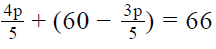• This is a linear equation with only 1 unknown, p. So, by solving this equation, we will be able to find the value of p
• Once we know the value of p, we can answer the question ‘Is p > 43?’
• So, Statement 2 also is sufficient to answer the question

Step 5: Analyze Both Statements Together (if needed)

Since we’ve already arrived at a unique answer in Steps 3 and 4, this step is not required

QUESTION: 3

### An investment of \$200,000 in an instrument that returns an annual rate of r percent compounded semi-annually grows to \$220,500 in one year. What is the value of r?

Solution:

Given:

• Principal = \$200,000
• Rate of return = r% per annum, compounded semi-annually
• Time = 1 year

= 2 half- years (that is, 2 periods of compounding)

• Amount = \$220,500

To Find: r = ?

Approach:

1. Since r is the annual rate of return but compounding happens every 6-months, the rate of return for 6 months = r/2 %
2. We are given the amount that the principal grows into in 2 periods of compounding. Using this information, we can find the rate of return for 6 months​

Working out: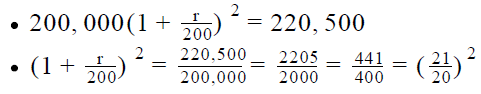• Upon taking square roots on both sides of this equation, 2 cases arise: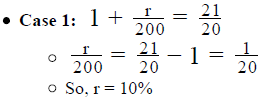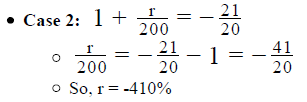• The negative value of r indicates degrowth in the principal (that is, instead of increasing, the invested principal decreased and the amount was less than the invested principal). However, this contradicts the given information (Amount is given to be \$220,500, which is greater than the invested principal).
• Therefore, this case is rejected
• Hence, we get a unique value of r = 10%
Looking at the answer choices, we see that the correct answer is Option C
QUESTION: 4

Lucy deposited \$62500 in an investment fund that provided 16 percent annual return compounded quarterly. If she made no other transactions with the fund, in how much time, in months, did her investment earn a total interest of \$5100?

Solution:

Given:

• Principal = \$62,500
• Rate of interest = 16% per annum, compounded quarterly
• So, rate of interest per quarter =
• Let the time period of investment be t quarters
• Interest earned in t quarters = \$5,100
• So, Amount = \$62,500 + \$5,100 = \$67,600

To Find: Time Period of Investment in Months

• = 3t months (since each quarter has 3 months)

Approach:

1. To find the value of 3t, we need to know the value of t, that is the number of quarters in which the investment earns the given interest
2. We are given the principal, and we have deduced the final amount and the quarterly rate of interest. We will use this information to find the value of

Working out: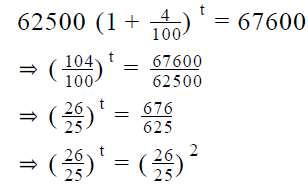• Since both sides of the above equation have the same base, the powers of the base on both sides of the equation will be equal as well.
• So, t = 2
• Remember that t denotes the number of quarters and the answer requires the number of months.
• The number of months in which the investment earns the given interest = 3t = 3*2 = 6 months
Looking at the answer choices, we see that the correct answer is Option D
QUESTION: 5

If \$200,000 invested at r percent per year, compounded quarterly, grow by \$88,000 in 6 months, what is the value of r?

Solution:

Given:

• Principal = \$200,000
• Rate of interest = r% per annum, compounded quarterly
• Time Period = 6 months
• = 2 quarters (that is, 2 periods of compounding)
• Growth in Principal = \$88,000
• So, Amount = \$200,000 + \$88,000 = \$288,000

To Find: r = ?

Approach:

1. Since r is the annual rate of return but compounding happens every quarter, the rate of return per quarter  = r/4 %
2. We are given the amount that the principal grows into in 2 periods of compounding. Using this information, we can find the rate of return per quarter

Working out: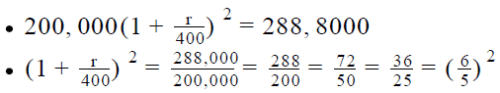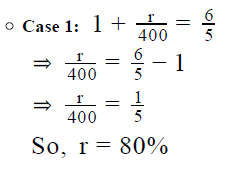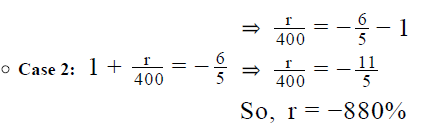• The negative value of r indicates degrowth in the principal (that is, instead of increasing, the invested principal decreased and the amount was less than the invested principal). However, this contradicts the given information (we’re given that the invested principal grew by \$88,000).
• Therefore, this case is rejected

So, r = 80%

Looking at the answer choices, we see that the correct answer is Option E

QUESTION: 6

Dan borrows a sum of \$10,000 from Lucy. Lucy charges Dan an interest of 10% p.a. compound interest, compounded annually. If
Dan pays back a total of \$13310 to Lucy, after how many years Dan pays back his entire debt?

Solution:

Given:

• Money borrowed by Dan (P) = \$10,000
• Rate of compound interest charged by Lucy (R) = 10% p.a., compounded annually
• Total amount paid back by Dan (A) = \$13,310

To Find:

Number of years Dan takes to pay his entire debt to Lucy.
Let the number of years be n.
Now we know that the formula for paying the total debt (which is paid at
a compounded interest) is given by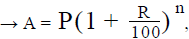where  R=10%p.a.; P=\$10,000 & A=\$13,310
So we know all the values to be used in the above formula except n → no. of years, which is to be calculated as desired in the question.

Approach:

• We know the values of A, R & P and also the total debt paid as given by the formula• Putting the values of knowns in the above formula, we will be able to calculate the value of n from the above formula.

Working out: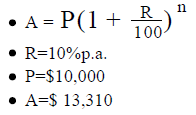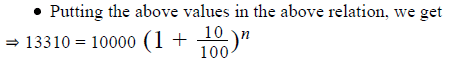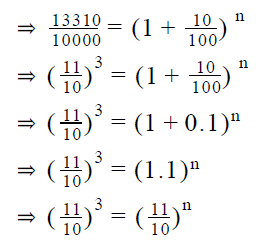Comparing both sides of the equation (as both are raised to sake basewe get
n=3 years.

• Hence number of years Dan takes to pay his entire debt is 3 years
• Hence answer option E is correct
QUESTION: 7

Jane and Nancy each saved \$2000 in the year 2014. If Jane saved 10% more in year 2015 than in year 2014, and Nancy saves 15%
less than what Jane saves in the year 2015, how much less money does Nancy save in year 2015 than in year 2014?

Solution:

Given:

• Let the money saved by Jane in year 2014 be SJ2014 . Given SJ2014 = \$2000
• Let the money saved by Nancy in year 2014 be SN2014 . Given SN2014 = \$2000
• Percentage more money saved by Jane in year 2015 than the money saved by Jane in year 2014 is 10%. Let this percentage be x. So we have → x = 10%
• Percentage less money saved by Nancy in year 2015 than the money saved by Jane in year 2015 is 15%. Let this percentage by y. So we have → y = 15%
• Let the money saved by Jane in year 2015 be SJ2015
• Let the money saved by Nancy in year 2015 be SN2015

To Find:

• We have to calculate how much less money does Nancy save in year 2015 than in year 2014.
• In other words, we have to calculate → SN2014 - SN2015
• Thus we somehow need to find the value of SN2015 to be able to calculate the difference in the savings of Nancy in the two years, as asked in the question. (We already are given the value of SN2014 = \$2000 )

Approach:

• In order to find → SN2015 , we will first work out on the savings made by Jane (SJ2015 ) in year 2015.
• To find the values of savings made by Jane in year 2015 (SJ2015 ), we will make use of the savings made by Jane in year 2014 and the percentage increase in savings (x) from year 2014 to year 2015, and use the relation ⇒ SJ2015 = SJ2014 + x*SJ2014
• After calculating the value of SJ2015 and from the percentage of less money (y) saved by Nancy in year 2015 from the money saved by Jane in year 2015, we will be able to calculate the savings of Nancy in year 2015 → SN2015 , by using the relation ⇒ SN2015 = SJ2015 - y*SJ2015
• Once we know the savings of Nancy in year 2015 → SN2015 , we can finally calculate the value of SN2015 - SN2014 , as desired in the question

Working out: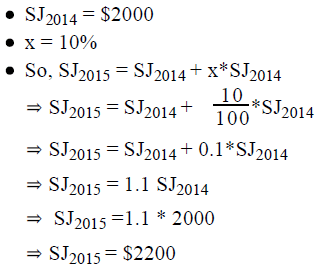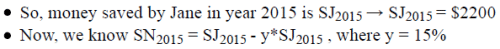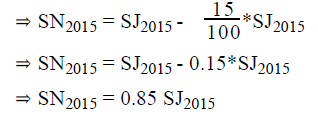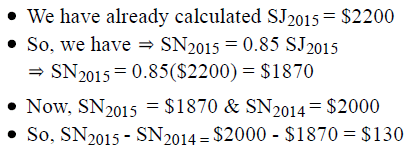• Hence the money saved by Nancy in year 2015 is \$130 less than the money saved by her in year 2014.
• Hence answer option D is correct.
QUESTION: 8

Roger distributed a total investment of \$1000 between mutual funds A and B and received a combined yearly return of 10 percent. How much did he invest in mutual fund A?

(1) Had he increased the share of mutual fund A in his total investment by 50 percent, he would have received a combined yearly return of 11 percent
(2) He received a return of 8 percent from mutual fund B

Solution:

Step 1 & 2: Understand Question and Draw Inference

Given:

• Total investment = \$1000
• Let investment in A = a dollars
• So, investment in B = 1000 – a dollars
• Total interest earned = 10% per annum
• = 10% of 1000 = \$100 per annum

To find :a = ?

• Let Investment A give a return of x percent and Investment B give a return of y percent.
• Since Total interest earned = \$100, we can write:
• Interest earned from Investment A + Interest earned from Investment B = 100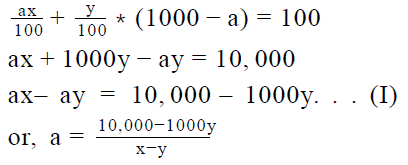• Thus, in order to know the value of a, we need to know the value of x and y.

Step 3 : Analyze Statement 1 independent

(1) Had he increased the share of mutual fund A in his total investment by 50 percent, he would have received a combined yearly return of 11 percent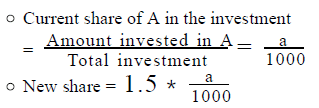• Note that the amount of total investment still remains \$1000 only. Only the allocation of this amount between investments A and B has changed
• So, new investment in A = 1.5a
• New investment in B = 1000 – 1.5a
• Given: Combined yearly return = 11% of \$1000 = \$110
• (Return from Investment A) + (Return from Investment B) = 110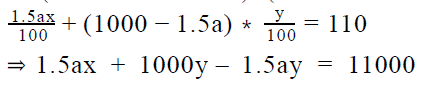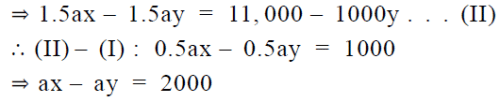• This equation has 3 unknowns. Therefore, it is not sufficient to determine a unique value of a

Step 4 : Analyze Statement 2 independent

2) He received a return of 8 percent from mutual fund B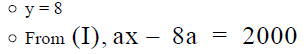This equation has 2 unknowns. Not sufficient to find a unique value of x.

Step 5: Analyze Both Statements Together (if needed)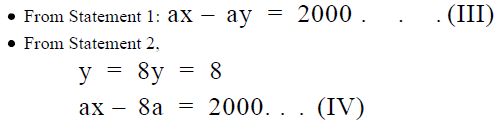• Substituting y = 8 in (III) we get:
• ax - 8a = 2000
• This is the same as Equation (IV)

So, even after both statements together, we cannot find a unique value of a.

QUESTION: 9

In the first year of a couple's marriage, the wife’s earnings were 40 percent of the combined earnings of the couple. The wife invested 40 percent of her earnings at an annual return of 5 percent and the husband invested 30 percent of his earnings at an annual return of 10 percent. In the second year of their marriage, the combined earnings of the couple increased by 10 percent and the wife’s earnings were five-sixths of her husband’s earnings. The wife invested 48 percent of her earnings and the husband invested 50 percent of his earnings in their respective investment instruments of the previous year. If the couple made no other investments and took out the interest earned in the first year at the beginning of the second year, by approximately what percent was the interest earned by the couple in the second year greater than the interest earned by the couple in the first year of their marriage? The interest income from the couple’s investments is not considered in their earnings.

Solution:

Given:

• Let the combined earnings of the couple in the 1 year be t.
• So, the given information can be tabulated as follows :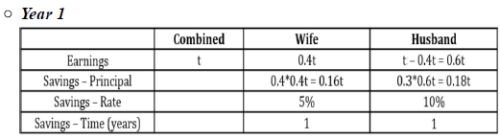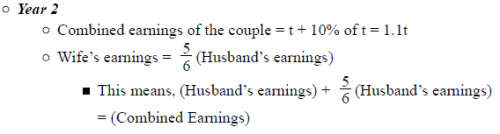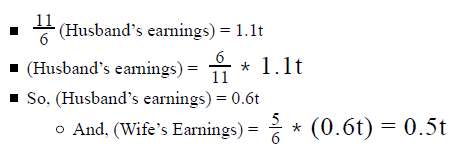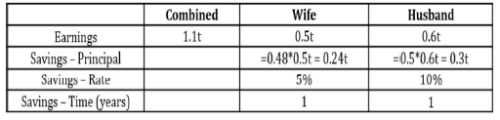To Find: Approximate percentage by which the 2nd year interest is greater than the 1st year interest

Approach:

1.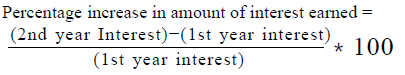• So, to answer the question, we need to find the (combined) 1 year interest and the (combined) 2nd year interest
2. For the 1st year, we know the savings principal, interest rate and time for both the wife’s investment and the husband’s investment. Therefore, we can easily find the interest earned by wife and by the husband. And, by adding these 2 interests, we’ll get the combined 1st year interest. The same process can be followed for the 2nd year as well. We have all the required information points.

Working out: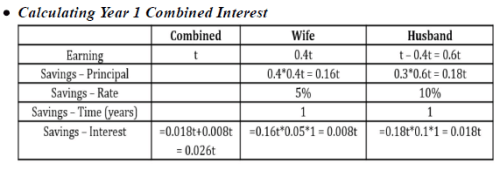• Calculating Year 2 Combined Interest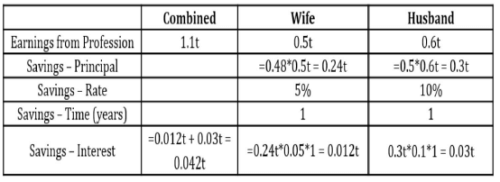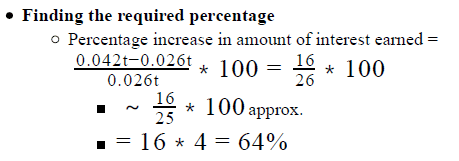• The actual value will be slightly less than 64% since the denominator 26 is greater than the denominator used for estimation. Looking at the answer choices, we see that the closest answer choice is 60%.

So, correct answer is Option D

QUESTION: 10

A person invests an equal amount of money in two investment schemes for two years. In the first investment scheme, he earns an
interest at 10% p.a. simple interest and in the second investment scheme he earns an interest at 10% p.a. compounded annually.
What is the difference in the interest earned under the two investment schemes at the end of the second year?

1. The amount the person invests in each scheme is \$10,000.
2. He earns an interest of \$1000 in the 1 scheme at the end of 1 year.
Solution:

Step 1 & 2: Understand Question and Draw Inference

Given

First  investment Scheme

• In the first scheme a person invests the amount at simple interest.
• We know, Simple Interest → S.I. =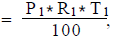, where P is the amount invested, P is the rate of interest for the investment, & T is the time during which the investment is made.
• Given to us is T =2 years, R =10% p.a., but we do not know the principal amount of 1 investment scheme (P ) over here.
• Since we do not know the value of P , we will not be able to calculate the amount of simple interest earned under the first investment scheme.

Second  investment Scheme

• In the second investment scheme he invests the amount at the compound interest.
• We know, in investment under compound interest, amount is given by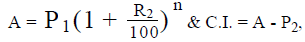where P2 is the amount invested, R2 is the rate of interest for the investment, & n is the time for which the investment is made.
• Given to us is n=2 years, R2 =10% p.a, but we do not know the principal amount (P2 ) of 2 investment scheme over here.
• Since we do not know the value of P , we will not be able to calculate the amount earned under the second investment scheme and thus will not be able to calculate the compound interest.
• Also Given is P1 = P2 (an equal amount of money is invested in both the schemes)

To Find:

• Difference in the interests earned under two investments scheme i.e. C.I. -S.I
• Since for knowing the values of S.I. and C.I. , we need to know the principal amount under two schemes, we need to analyse the statements further to be able to calculate the difference in the interests under two schemes.

Step 3 : Analyze Statement 1 independent

Statement 1

• The amount the person invests in each scheme is \$10,000.
• So we know the Principal amount invested under each scheme or we are given the value of P1 & P2 , we will be able to calculate the C.I. and S.I. as already established above.
• Thus statement 1 will be sufficient to answer the question.

Step 4 : Analyze Statement 2 independent

Statement 2:

• He earns an interest of \$1000 in the 1 scheme at the end of 1 year.
• Now the first scheme is an investment made under Simple interest.
• By the formula of S.I., we have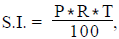, where S.I. =\$1000, R → 10% & T=1 year, we will be able to calculate the value of P.
• The P calculated above will be equal to P i.e. the investment under the first scheme, which in turn is also equal to P2.
• Now calculating the values of P & P2, we will be able to calculate the C.I. and S.I. as already established above.
• Thus statement 2 will be sufficient to answer the question.

Step 5: Analyze Both Statements Together (if needed)

• Since from statement 1 and statement 2, we are able to arrive at a unique answer, combining and analysing statements together is not required.
• Hence the correct answer is option D
QUESTION: 11

Rosy invested x dollars at a simple interest of y percent per annum for n years with bank A and x/2 dollars at a simple interest of z percent per annum for m years with bank B. Was the interest generated at the end of the respective tenures of investments greater in bank A than in bank B?

1) The amount received at the end of the tenure from bank A was less than twice the amount received from bank B.
2) Had the rate of simple interest offered by bank B equal to the rate of simple interest offered by Bank A, it would have taken bank B 2m years to generate the same amount of interest as it did in m years with z percent per annum rate of simple interest.

Solution:

Step 1 & 2: Understand Question and Draw Inference

Bank A

• Principal invested = x dollars
• Interest rate = y% per annum simple interest
• Time period = n years

Bank B

• Principal invested = x dollars
• Interest rate = z% per annum simple interest
• Time Period = m years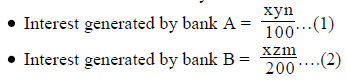To Find: Is Interest generated in the investment in Bank A > Interest generated in the investment in Bank B?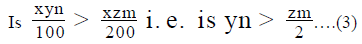Step 3 : Analyze Statement 1 independent

1. The amount received at the end of the tenure from bank A was less than twice the amount received from bank B.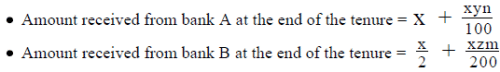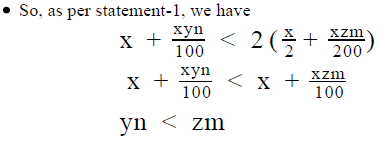However, we cannot say for sure if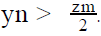Hence insufficient to answer.

Step 4 : Analyze Statement 2 independent

2. Had the rate of simple interest offered by bank B equal to the rate of simple interest offered by Bank A, it would have taken bank B 2m years to generate the same amount of interest as it did in m years with z percent per annum rate of simple interest.

•  As it takes bank B more years to generate the same interest, bank A’s interest is being equated to bank B’s interest.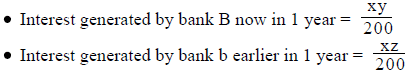• As bank B will take 2m years to generate the same interest as it generated earlier, we can write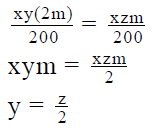Putting this in (3), the question simplifies to: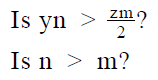However we do not know for sure if n > m. Insufficient to answer.

Step 5: Analyze Both Statements Together (if needed)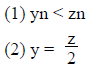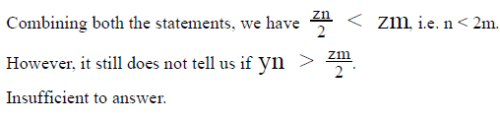QUESTION: 12

A moneylender lent \$2000 to Ricky with the condition that Ricky will pay back 5 percent of this amount every half year. Till Ricky repaid the original loan amount (principal) completely, the moneylender charged an interest equal to 5 percent per quarter on the original loan amount. Ricky always made interest payments and principal repayments on time. (For example, at the end of the first quarter, Ricky paid the moneylender 5 percent of \$2000 as interest and at the end of 6 months, Ricky paid the money lender 5 percent of \$2000 as interest and 5 percent of \$2000 as principal repayment). What was the total amount in dollars that Ricky paid to the moneylender?

Solution:

Given:

• Amount lent = \$2000
• Repayment = 5% of the amount lent every 6 months
• Repayment amount = 5% of \$2000 = \$100 every 6 months
• Interest charged = 5% per every 3 months on \$2000
• Interest charged = 5% of \$2000 = \$100 every 3 months

To Find: Total amount paid to the money lender?

Approach:

1. Total amount paid to the moneylender = Principal Repaid + Interest paid
• We know Principal Repaid = \$2000
2. For finding the total interest paid, we need to find the time period for which the interest was paid
• Since the rate of interest is given per quarter, we need to express the time period too in terms of quarters.
3. Now, the time period for which the interest was paid is equal to the time for which Repayments of the principal were done.
• As \$100 of the principal was repaid every 6 months (that is, half-year), we can find the number of half-years takentime taken to pay the total principal
• (Number of half-years)=(Total Principal to be Repaid)/(Repayment done per half-year)
• So, (Number of quarters taken to complete repayment) = 2(Number of half-years)

Working out:

1. Total principal to be paid = \$2000
• Principal paid every half year = \$100
• So, it will take half years to pay back \$2000
2. 20 half years = 40 quarters
• Interest paid in 1 quarter = \$100
• Interest paid in 40 quarters = 40 * 100 = \$4000
3. Total amount paid = \$2000 + \$4000 = \$6000

Hence, Ricky paid a total amount of \$6000 to the money lender including the principal and the interest.

QUESTION: 13

What dollar amount invested at the rate of 20 percent per annum compounded annually for 2 years yields an interest of \$176?

Solution:

Given:

• Let the amount invested, P, be equal to x dollars
• Rate of interest, R = 20% per annum compounded annually
• Time period, n = 2 years
• Interest, CI = \$176

To Find: Value of x?

Approach:

1. We know that Compound Interest,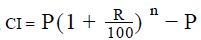• We will put the values of the different entities in the above equation to calculate the value of x

Working out: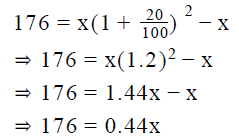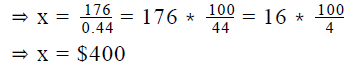So, the amount invested was \$400.

QUESTION: 14

Sonya invested \$20,000 in a mutual fund scheme, which calculated and reinvested the interest earned in the scheme annually. She received a return of 10 percent in the first year, 20 percent in the second year and 5 percent in the third year. By what amount, in dollars, did her money grow in the three years?

Solution:

Given:

• Principal = \$20,000
• Rate of Interest:
• 1st year: 10%
• 2nd year: 20%
• 3rd year: 5%

To Find: The Amount by which her money grew in 3 years

Approach:

1. Amount by which her money grew = (Amount at the end of 3 year) – (Principal)
• = (Amount at the end of 3rd year) – 20,000
2. To calculate the amount at the end of 3rd year, we will have to consider the successive annual % increases in the invested amount

Working out: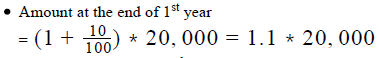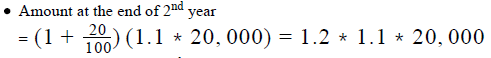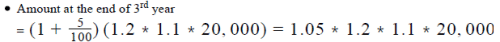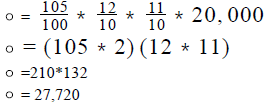• Remember that we are not asked about the amount at the end of 3rd year, but about the amount by which her money grew in 3 years
• Growth in her money = 27,720 - 20,000 = 7,720

So, the correct answer is Option B

QUESTION: 15

Martina invested \$1000 for a period of 17 years in a bank that paid variable rates of interest. For the first 2 years, the bank paid an annual interest rate of x percent compounded annually and for the next 5 years, the bank paid a simple interest rate of 12 percent per annum on the compounded amount at the end of the second year. If for the remaining years, Martina earned a total simple interest of \$1694 on the compounded amount at the end of the second year, at a rate of 14 percent per annum, by approximately what percentage of the invested amount was the total simple interest earned greater than the compound interest earned?

Solution:

Given:

• Amount invested = \$1000
• Time period of investment = 17 years
• Rate of interest
• 0- 2 years = x% p.a. compounded annually
• 3-7 years = 12% p.a. simple interest
• 8-17 years = 14% p.a. simple interest
• Total interest earned for the time period 8 – 17 years = \$1694 on the amount at the end of the 2 year.

To Find:

By what percentage of the invested amount was the total simple interest earned greater than the compound interest earned?

• Let the total simple interest earned be SI and the total compound interest earned be CI.
• SO, the difference between the interest earned = SI – CI.
• We need to find the value of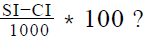• So, we need to find the value of SI – CI or the values of SI and CI

Approach:

1. Total Simple interest earned = \$1694 + interest earned from 3-7 years at 12% p.a.
• We know that Simple interest =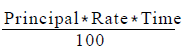• To find the simple interest earned from 3- 7 years we need to know the principal on which the interest rate of 12% p.a. was applied, i.e. the principal at the end of the 2 year.
2. Compound interest earned = Interest earned in 0-2 years at x% p.a.
• Compound Interest = , where principal Invested = \$1000
• So, to calculate the compound interest, we need to know the final amount at the end of the 2nd year,
• Let the amount at the end of 2nd year be P
3. As we are given that principal P at 14% p.a. simple interest earned a total interest of \$1694, we can find the principal P using the formula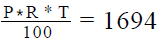Working out:

1. For the time period 8-17 years

a. Principal = P
b. Rate of Interest = 14% p.a.
c. Time period = 17 - 8 + 1 = 10 years

d.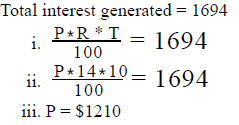2. For the time period 3-7 years
a. Principal = \$1210
b. Rate of interest = 12% p.a.
c. Time period = 5 years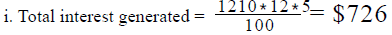3. Total simple interest earned = \$1694 + \$726 = \$2420……..(1)
4. For the time period 0-2 years
a. Principal = \$1000
b. Amount = P = \$1210
c. Total compound interest earned = \$1210 - \$1000 = \$210…….(2)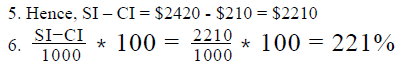QUESTION: 16

Roxie invested \$2000 in an account that yielded a fixed rate of annual return compounded annually throughout the duration of
investment. If she earned a total interest of \$4000 in 6 years, after how many years of investment did she earn a total interest of
\$52000?

Solution:

Given:

• P = \$2000
• Let the rate of return be R% per annum
• Total compound interest earned in 6 years = \$4000
• So, Amount after 6 years = Principal \$2000 + Interest \$4000 = \$6000

To Find: Number of Years after which she earned a total interest of \$52,000

Approach:

1. Let the total interest be \$52,000 after x years of investment
• So, Amount after x years = Principal \$2,000 + Interest \$52,000 = \$54,000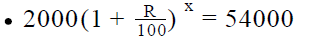• From this equation, it’s clear that to find the value of x, we need to find the value of R.

2. We’re given another detail of this investment. In 6 years of time, the principal \$2000 had grown to an amount of \$6000. Using this, we can find the value of R.

Working out: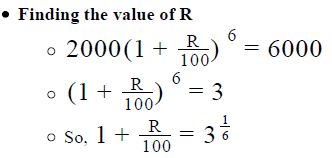• Note: the reason why we stopped at this point and didn’t calculate till the value of R is that the equation in which R is going to be used (see the equation right under the heading ‘Finding the value of x’) contains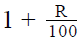only. So, it will
be a wastage of time if we first solved the above equation till we got the exact value of R, and then, after substituting that
value of R in the below equation, spent time in calculating the value of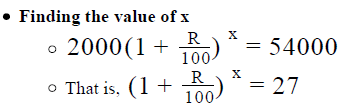Substituting the above found value ofin the above  equation: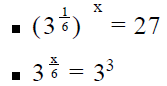• Since the base is equal on both sides of the equation, the powers must be equal as well: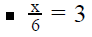• So, x = 18 years

Looking at the answer choices, we see that the correct answer is Option A

QUESTION: 17

A \$200 investment at x percent per annum and a \$500 investment at y percent per annum have a combined yearly return of 10 percent of the total of the two investments. If \$400 is invested at x percent and \$600 is invested at y percent per annum to give a combined yearly return of 9.2 percent of the total of the two investments, what will be the combined percentage yearly return of the total investment if \$100 each is invested at x percent per annum and y percent per annum respectively?

Solution:

Given:

• Case-I
• Principal , P = \$200
• Rate of interest = x% p.a.
• Principal, P = \$500
• Rate of Interest = y% p.a.
• Combined yearly return = 10%
• Case-II
• Principal, P = \$400
• Rate of interest = x% p.a.
• Principal, P = \$600
• Rate of Interest = y% p.a.
• Combined yearly return = 9.2%

To Find: Combined yearly return if \$100 each is invested at x% p.a. and y% p.a.?

• Let the combined yearly return be R%
• So, Return on \$200 invested at R% p.a. = Return on \$100 at x% p.a. + Return on \$100 at y% p.a.
•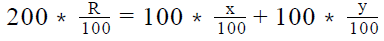Approach:

1. For finding the value of R, we need to find the value of x and y.
2. We are given two cases in which the principals are invested at x% and y% per annum and we are also given the combined yearly return for both the cases
• The combined yearly return is equal to the sum of the returns of the individual investments
• Writing the combined yearly return equations for each of the case will give us an equation in x and y
3. We will solve both the equations to get the value of x and y, which will be used to calculate the yearly return on \$100 each invested at x% and y% p.a.

Working out:

1. Case-I
• As the combined yearly return is equal to the sum of the returns of individual investments, we can write the following equation
•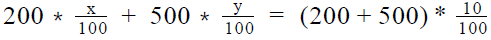• 2x +5y = 70……………..(1)

2. Case-II

• As the combined yearly return is equal to the sum of the returns of individual investments, we can write the following equation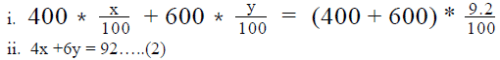3. Solving (1) and (2), we have y = 12 and x = 5
4. Hence, combined yearly return on investment of \$100 each at x% and y% can be calculated as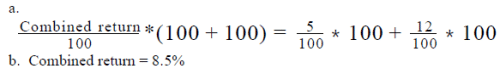QUESTION: 18

Petro invested x dollars each in scheme A and scheme B for two years. Scheme A offered a simple interest rate of 10 percent per
annum while scheme B offered an interest rate of 10 percent per annum compounded annually. If the amount received by Petro at
the end of two years in scheme B was \$63 greater than the amount received from scheme A, how much in dollars did he invest in
scheme A?

Solution:

Given:

• Scheme A
• Principal PA invested = x dollars
• Rate of interest, RA = 10% p.a. Simple Interest
• Investment time, TA = 2 years
• Amount received after 2 years = AA
• Scheme B
• Principal PB invested = x dollars
• Rate of interest, RB = 10% p.a. compounded annually
• Investment time, TB = 2 years
• AB - AA = 63

To Find: Value of x?

Approach:

• For finding the value of x, we need to form an equation in x.
• As we are given that x dollars each was invested in schemes A and B, we
• can calculate the amount received from schemes A and B using the following formula: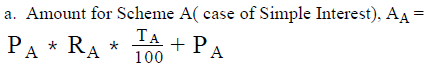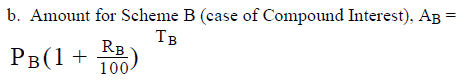3. We can then use the relation AB- AA = 63 to formulate an equation in x.

Working out: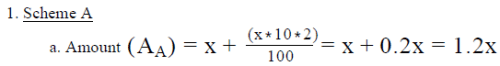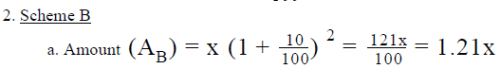3. Substituting the values of A and A in the relation A - A = 63, we have
a. 1.21x - 1.20x = 63
b x = \$6300

Hence, Pedro invested \$6300 in scheme A

QUESTION: 19

Novak invested \$5000 in scheme A for 4 years, which gave a fixed rate of return each year. From the second year of this investment, the interest earned on scheme A in the previous year was reinvested annually in scheme B, whose annual rate of interest, compounded annually, varied with the performance of scheme B in that particular year. If the total interest earned from both the schemes in the first 3 years was \$500, \$550 and \$710 respectively and scheme B offered an interest rate of 5 percent per annum in the fourth year, what was the total interest in dollars that was earned in the fourth year from both the schemes?

Solution:

Given:

• Scheme A
• Principal invested = \$5000
• Time period = 4 years
• Offered a fixed rate of return
• Scheme B
• Each year investment = Interest generated from scheme A in
• pervious year
• Rate of interest in 4 year = 5% p.a.
• Total Interest generated
• Year 1 = \$500
• Year 2 = \$550
• Year 3 = \$710

To Find: Total interest earned in the 4 year from both the schemes?

Approach:

1. For finding the total interest earned in the 4 year, we need to find the interest earned from both the schemes.
2. For finding the interest earned, we need to find the principal and the rate of interest of both the schemes.
3. Scheme A
• As the interest generated in every year is taken out, the principal in scheme A will not change. So, the principal in scheme A in 4 year = \$5000
• As scheme A offers, a fixed rate of return every year, if we can find the rate of interest in any one of the year, we will be able to find the rate of interest offered in the 4th year
• In the 1st year, the total interested generated will be equal to the interest generated by scheme A. As we know the principal and the interest generated for the 1st year, we can find the rate of interest offered by scheme A
4. Scheme B
• As we are given the interest rate offered by scheme B in the 4th year, we only need to find the principal.
• (The principal of scheme B in the 4th year) = (The interest generated by scheme A in the 1st + 2nd +3rd year) + (The interest generated in scheme B in the 2nd and the 3rd year)
• As we already know the interest generated by scheme A in each year(which is constant), we can find the sum of the interest generated by scheme A in 3 years.
• Also, since we are given the total interest generated by the schemes in the 1st , 2nd and the 3rd year, we can find the interest generated by scheme B in each year

Working out:

1. Interest generated by scheme A = \$500 each year
• Since the interest generated in the 1 year will exclusively come from scheme A
2. As scheme A generates a fixed interest every year, it will generate an interest of \$500 every year
3. Also, as \$500 was received as interest in year 1, the rate of interest for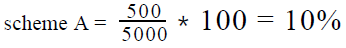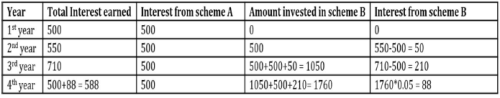4. Principal of scheme B will grow each year by the interest generated in scheme A as well as the interest generated in scheme B

• Hence, the principal of scheme B at the start of the 4 year = 500 * 3 + 50 + 210 = 1760

5. So, the interest generated in 4 year:
a. Scheme A = \$500
b. Scheme B = 5% of 1760 = \$88
c. Total interest = 500 + 88 = \$588Use Code STAYHOME200 and get INR 200 additional OFF Use Coupon Code